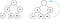# The curious case of heaps

At first glance, one might not be able to distinguish between the two trees above, because you know, they all look similar. However, on getting a closer look, the nodes seem to be ordered in some logical way. In the figure above, the tree on the left is a Binary Search Tree and the one on the right is a Binary Heap, specifically a Min Heap. Both of them are binary trees, but each follows a different set of rules.

A binary heap is simply a binary tree that obeys the following rules -

• The tree must be complete — each node in the tree must have two children in all levels except the last one, and the nodes in the last level must be filled from left to right. This ensures that the tree is balanced and enables insertions and deletions to be done in O(height of the tree) which is equal to O(log(number of nodes)) or O(log n).
• The parent and child nodes of the tree must satisfy the heap property. In a max heap, the parent’s key must be greater than or equal to the keys of its children, and vice-versa for a Min Heap.

Heaps find their application in a variety of algorithms. A Priority Queue is an abstract data type implemented using a heap. Priority queues are used in several graph algorithms such as Dijkstra and Prim’s MST. Heaps are also used in their own sorting algorithm — heapsort, which uses a heap to sort integers in-place and in O(nlogn) time.

# Array representation of a heap

Like a binary tree, binary heaps can be represented using an array. If we start from index 0, for every node at index i, its left child would be at index 2*i+1 and right child at index 2*i+2. The node’s parent would be at floor((i-1)/2). The array representation of the following heap is as shown below.

# Insertion

While inserting elements into a heap, there are two things to be taken care of — the heap must remain balanced and the heap property mustn't be violated. For example, if we wanted to insert 1 into the min-heap shown below, we would have to insert it in the last level to preserve the first rule. But doing so would violate the second rule.

So we are now faced with the task of bringing the element up into the tree so that we can satisfy all the rules. We do that by swapping the element with its parent if the heap property is being violated. We repeat the process until we have a rule-abiding heap :p.

The time complexity of this operation would be O(h), where h is the height of the heap. Since the heap is balanced, we can rewrite it as O(logn), where n is the number of nodes in the heap.

# Get Min/Max element in the heap

One huge advantage of heaps is that they allow you to get the maximum or minimum element in the heap quickly. But once that element has been removed, we have the task of choosing a new node to take its place. And the chosen node mustn't violate any of the rules.

We do this by picking the last element at the last level to be the new root. But alas, we might end up violating the second rule. So we have to find a way of restoring order withing the heap :p.

In the insertion process, we compared the newly inserted node with a single node(its parent) and swapped the node up to its correct position.

In this case, we have to compare the newly inserted node with two nodes(its children) and pick the smaller one to swap it with. This way the new node is swapped down to its correct position. This is basically what the heapify() function does. Since the heap is balanced, this can be achieved in O(logn) time.getMin() operation on a Min Heap — the last node replaces the root

For example, the getMin() function is called on the heap shown below. After the root element has been extracted, the last element in the min-heap replaces it. This ends up violating the heap property, and its no longer a min-heap.

To convert it into a min-heap, the min_heapify() function is called on the root. The root node is compared with the child nodes and is swapped with the appropriate node(in this case the one with a smaller key, since its a Min Heap). The function recursively swaps the incorrect node down to its correct position.

# Heap Sort

The getMax() property can easily remove the largest element from a heap, this can now be used to sort an array in ascending order. To sort an array using heapsort, there are two steps to be carried out—

1. We have to build a max heap from the array. To build a max heap from an unsorted array, we would have to call max_heapify() for every internal(non-leaf) node. This step has a time complexity of O(n).
2. Then we repeatedly extract the max element and swap it with the last element in the array. Since this might violate the heap property again, we max_heapify the remaining heap. The size of the heap now reduces by one. This process is repeated until a single element is left in the max-heap. In this way, we can sort the array in-place and in O(nlogn).